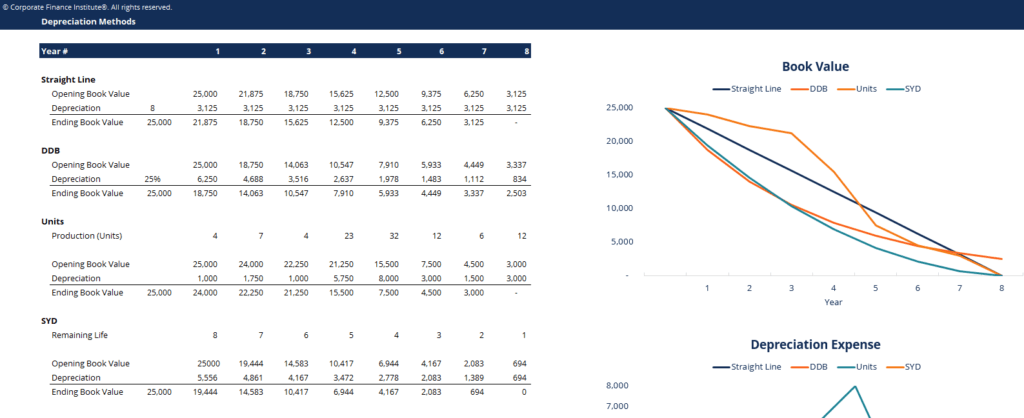# Depreciation Methods Template

## Depreciation Methods Template

This depreciation methods template will show you the calculation of depreciation expenses using four types of commonly use depreciation methods.

Below is a preview of the depreciation methods template:### Depreciation Methods Template

There are several types of depreciation expense and different formulas for determining the book value of an asset. The most common depreciation methods include:

1. Straight-line
2. Double declining balance
3. Units of production
4. Sum of years digits

Depreciation expense is used in accounting to allocate the cost of a tangible asset over its useful life. In other words, it is the reduction of value in an asset over time due to usage, wear and tear, obsolescence.  The four main depreciation methods mentioned will be explained in detail below.

### #1 Straight-Line Depreciation Method

Depreciation Expense = (Cost – Salvage value) / Useful life

### #2 Double Declining Balance Depreciation Method

Periodic Depreciation Expense = Beginning book value x Rate of depreciation

### #3 Units of Production Depreciation Method

Depreciation Expense = (Number of units produced / Life in number of units) x (Cost – Salvage value)

### #4 Sum-of-the-Years-Digits Depreciation Method

Depreciation Expense = (Remaining life / Sum of the years digits) x (Cost – Salvage value)

### More Free Templates

For more resources, check out our business templates library to download numerous free Excel modeling, PowerPoint presentation and Word document templates.

• Excel Modeling Templates
• PowerPoint Presentation Templates
• Transaction Document Templates

### Financial Analyst Certification

Become a certified Financial Modeling and Valuation Analyst (FMVA)® by completing CFI’s online financial modeling classes and training program!